# Introduction to Graphs Graphs an overview vertices Graphs

• Slides: 31
Download presentationIntroduction to Graphs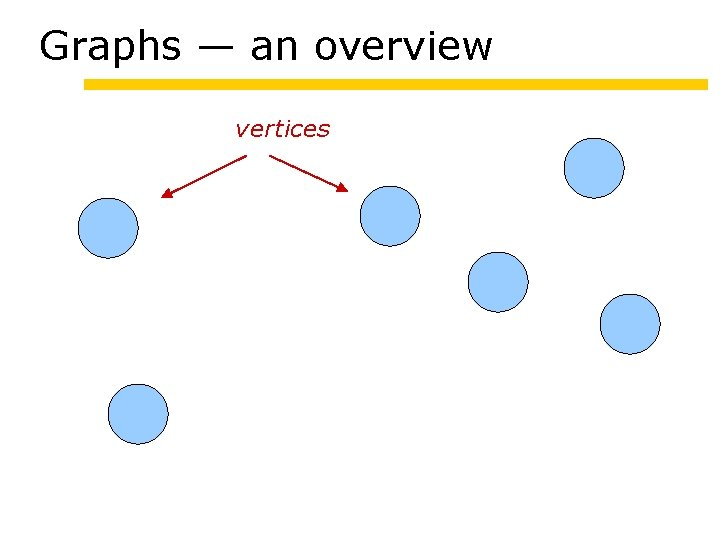Graphs — an overview vertices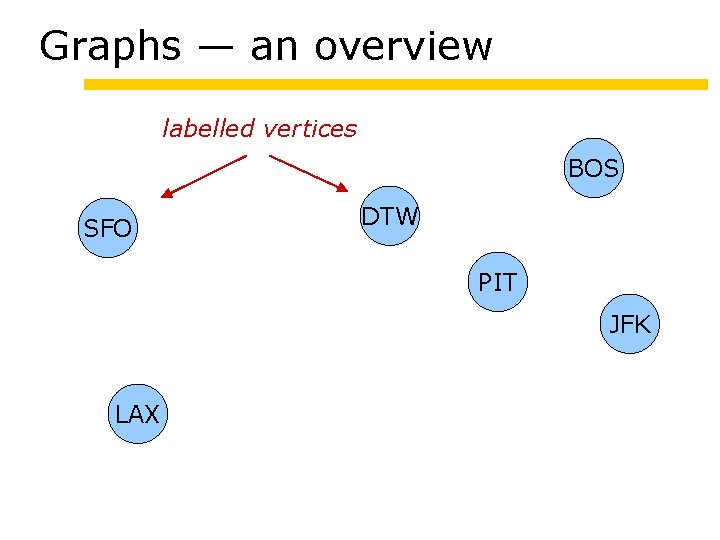Graphs — an overview labelled vertices BOS SFO DTW PIT JFK LAX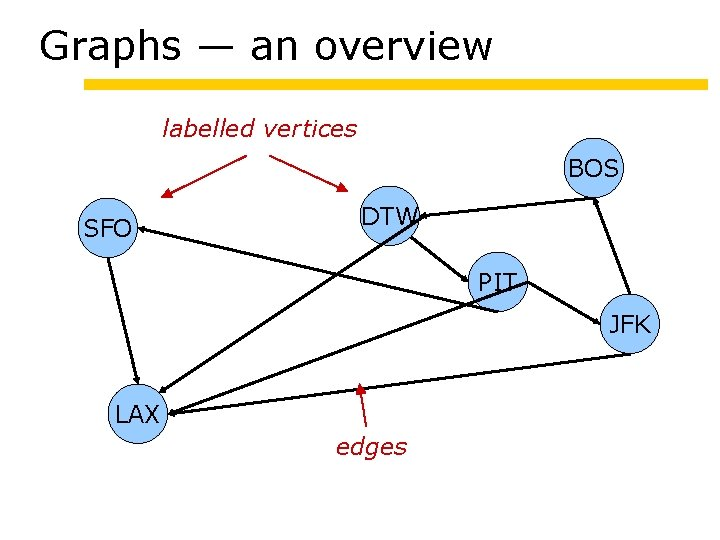Graphs — an overview labelled vertices BOS SFO DTW PIT JFK LAX edgesGraphs — an overview labelled vertices BOS SFO DTW PIT JFK LAX undirected edgesGraphs — an overview labelled vertices 618 SFO DTW 2273 211 190 PIT 1987 344 LAX BOS 318 JFK 2145 2462 labelled edgesTerminology - vertices (nodes, points) - edges (arcs, lines) directed or undirected multiple or single loops - vertex labels - edge labels G = (V, E) or G = (V, E, lab)Edges - directed (x, y) or just xy x is the source and y the target of the edge - undirected {x, y} or just xy Note that {x, x} means: undirected loop at x. - edge is incident upon vertex x and yDegrees - directed out-degree of x: the number of edges (x, y) in-degree of x: the number of edges (y, x) degree: sum of in-degree and out-degree - undirected degree of x: the number of edges {x, y} Degrees are often important in determining the running time of an algorithm.Graphs are Everywhere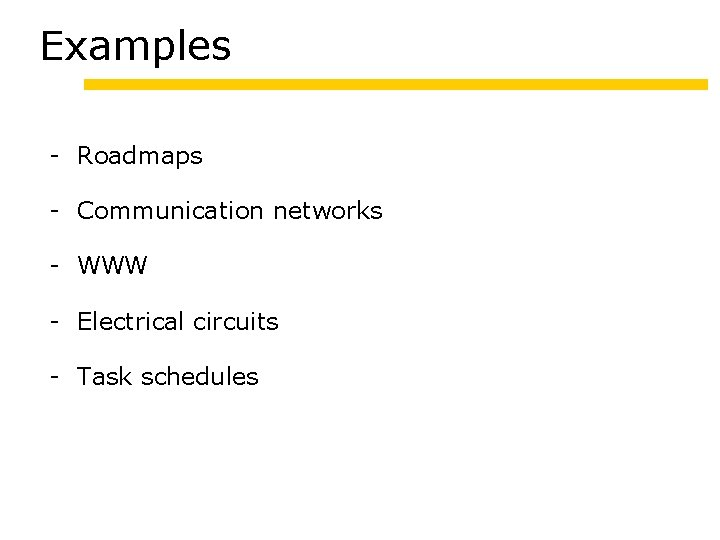Examples - Roadmaps - Communication networks - WWW - Electrical circuits - Task schedulesGraphs as models Physical objects are often modeled by meshes, which are a particular kind of graph structure. By Jonathan ShewchukWeb Graph <href …> <href …> Web Pages are nodes (vertices) HTML references are links (edges)Relationship graphs Graphs are also used to model relationships among entities. Scheduling and resource constraints. Inheritance hierarchies. 15 -113 15 -151 15 -212 15 -251 15 -213 15 -312 15 -411 15 -462 15 -412 15 -451More Generally Suppose we have a system with a collection of possible configurations. Suppose further that a configuration can change into a next configuration (transition, non-deterministic). Model by a graph G = ( configurations, transitions ) Evolution of the system corresponds to a path in the graph.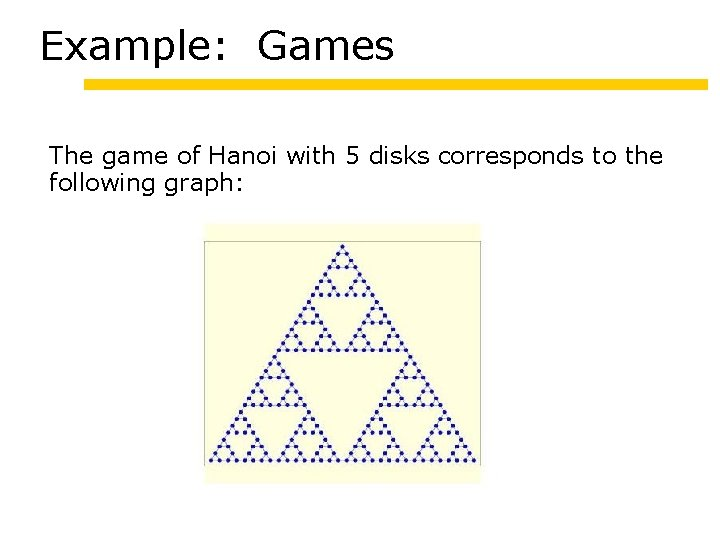Example: Games The game of Hanoi with 5 disks corresponds to the following graph:Solving a Game A solution is just a path in the graph: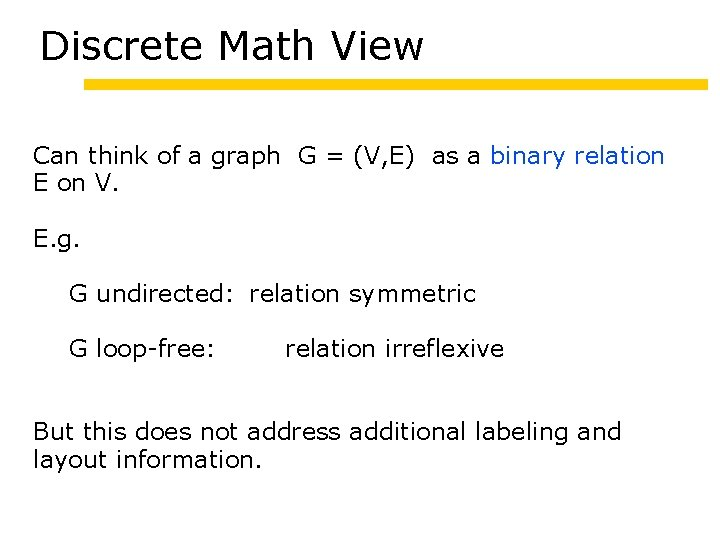Discrete Math View Can think of a graph G = (V, E) as a binary relation E on V. E. g. G undirected: relation symmetric G loop-free: relation irreflexive But this does not address additional labeling and layout information.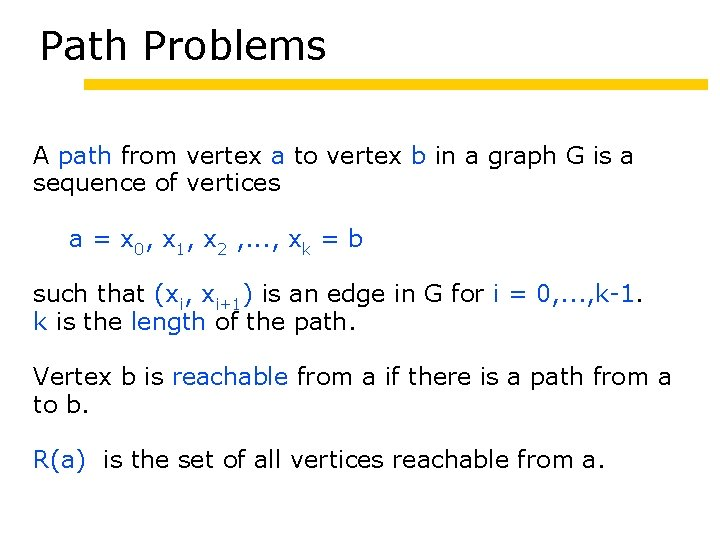Path Problems A path from vertex a to vertex b in a graph G is a sequence of vertices a = x 0, x 1, x 2 , . . . , xk = b such that (xi, xi+1) is an edge in G for i = 0, . . . , k-1. k is the length of the path. Vertex b is reachable from a if there is a path from a to b. R(a) is the set of all vertices reachable from a.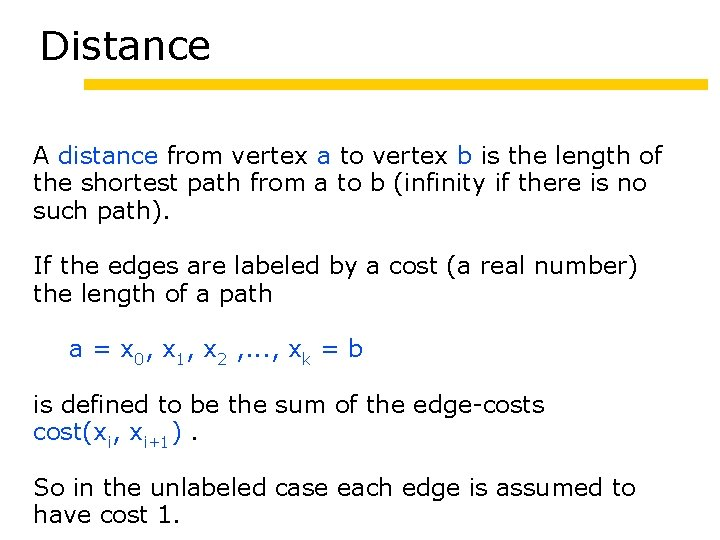Distance A distance from vertex a to vertex b is the length of the shortest path from a to b (infinity if there is no such path). If the edges are labeled by a cost (a real number) the length of a path a = x 0, x 1, x 2 , . . . , xk = b is defined to be the sum of the edge-costs cost(xi, xi+1). So in the unlabeled case each edge is assumed to have cost 1.Exercise Find a good way to compute the distance of any two Hanoi configurations.Connectivity A graph G is connected if R(a) = V for all vertices a. For an undirected graph this is equivalent to R(a) = V for some vertex a. A connected component of a ugraph G is a set C that is connected (meaning R(a) = C for all a in C) and that is a maximal such. For digraphs the situation is more complicated, postpone.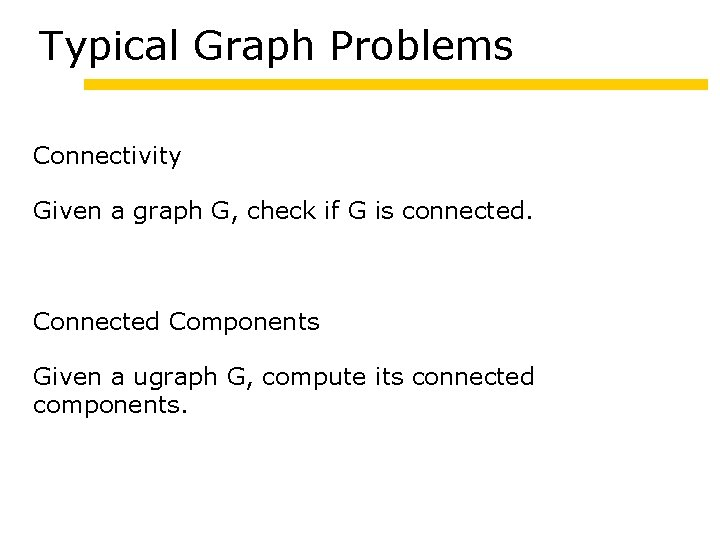Typical Graph Problems Connectivity Given a graph G, check if G is connected. Connected Components Given a ugraph G, compute its connected components.Typical Graph Problems Shortest Path Given a graph G and vertices a and b, find a shortest path from a to b. Distance Given a graph G, compute the distance between any pair of vertices.Representing Graphs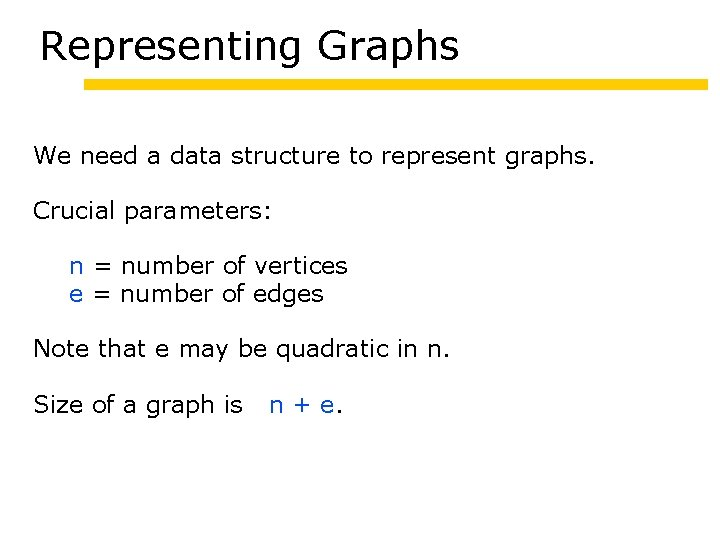Representing Graphs We need a data structure to represent graphs. Crucial parameters: n = number of vertices e = number of edges Note that e may be quadratic in n. Size of a graph is n + e.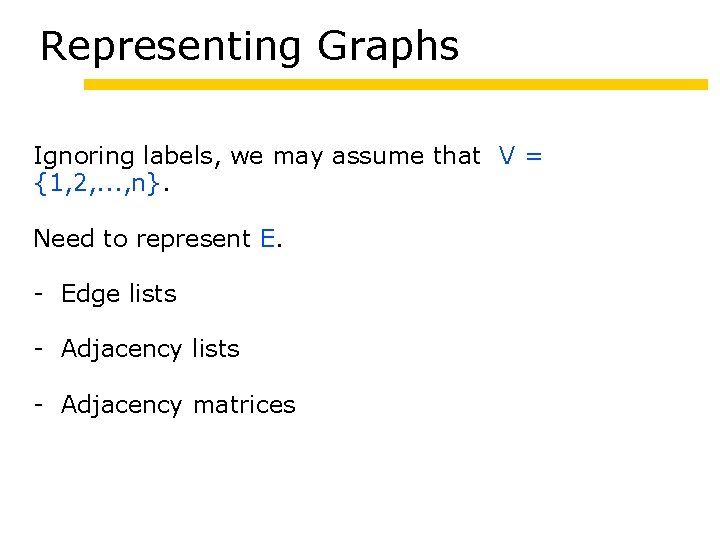Representing Graphs Ignoring labels, we may assume that V = {1, 2, . . . , n}. Need to represent E. - Edge lists - Adjacency matricesSupporting Operations We need to be able to perform operations such as the following: - insert/delete a vertex insert/delete an edge check whether (x, y) is an edge given x, enumerate its neighbors y Enumerating neighbors is crucial in many graph algorithms. Example: Compute degrees.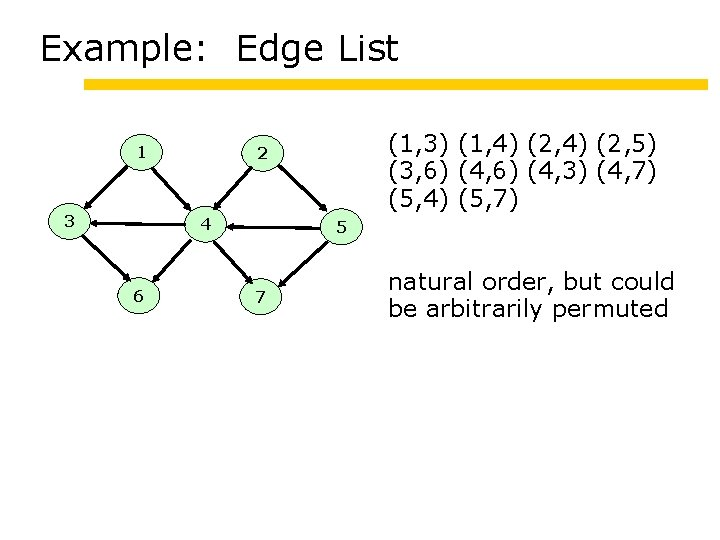Example: Edge List 1 3 4 6 (1, 3) (1, 4) (2, 5) (3, 6) (4, 3) (4, 7) (5, 4) (5, 7) 2 5 7 natural order, but could be arbitrarily permuted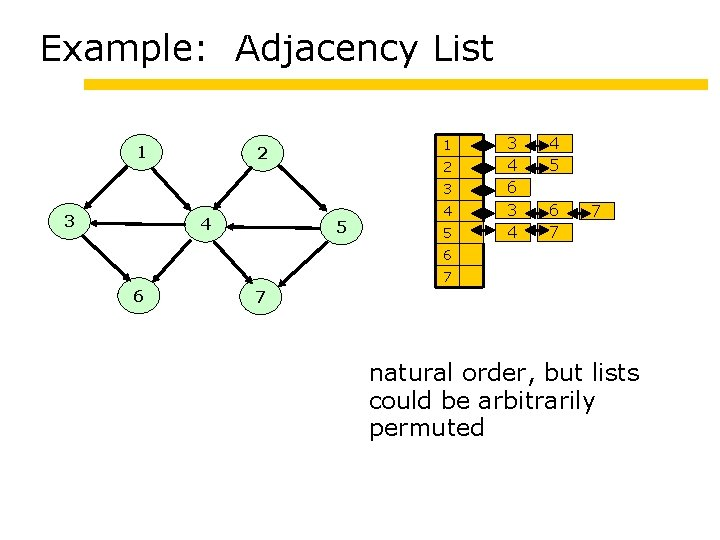Example: Adjacency List 1 1 2 2 3 3 4 5 3 4 6 3 4 4 5 6 7 7 6 7 natural order, but lists could be arbitrarily permuted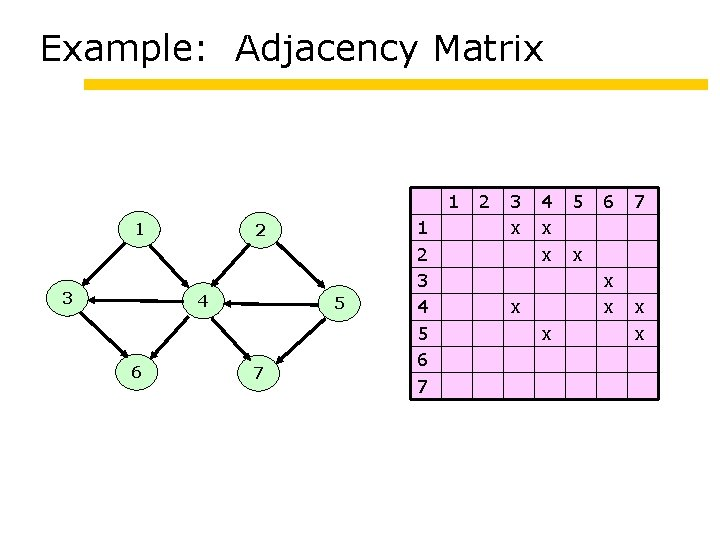Example: Adjacency Matrix 1 1 3 2 4 6 5 7 1 2 3 4 5 6 7 2 3 x 4 x x 5 6 x x x 7 x x